# Resistor Symbols

Resistor symbols of electrical & electronic circuit diagram - resistor, potentiometer, variable resistor.

## Table of resistor symbols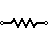Resistor (IEEE) Resistor reduces the current flow.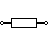Resistor (IEC)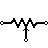Potentiometer (IEEE) Adjustable resistor - has 3 terminals.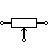Potentiometer (IEC)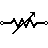Variable Resistor / Rheostat (IEEE) Adjustable resistor - has 2 terminals.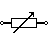Variable Resistor / Rheostat (IEC)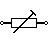Trimmer Resistor Preset resistor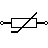Thermistor Thermal resistor - change resistance when temperature changes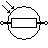Photoresistor / Light dependent resistor (LDR) Photo-resistor - change resistance with light intensity change

Capacitor symbols ►